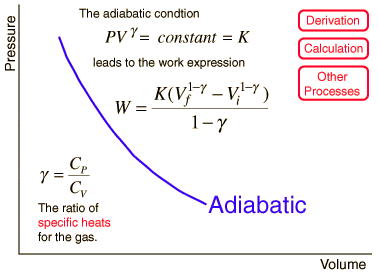TheBeesKnees

## Homework Statement

During an adiabatic process, the temperature of 5.50 moles of a monatomic idea gas drops from 495 C to 215 C. For this gas, find (a) the work it does, (b) the heat it exchanges with its surroundings, and (c) the change in its internal energy

## Homework Equations

I know work is P(delta V)
PV1=nRT1 and
PV2=nRT2 so if I subtract to get the change,
P(deltaV)=nR(delta T)
P(deltaV)=5.50(8.31)(280)
P(deltaV)= 12797.4 Joules.
But my textbook says this isn't the answer.
Where did I go wrong?

I know the answer for b is zero, since that's the definition of an adiabatic process

TheBeesKnees
Oh yeah, and the answer to part c must be the opposite sign but same magnitude of the answer to a.

physixguru
What is the work done during an ADIABATIC process... ?

Is it really (P deltaV) ?

Have a look...Last edited:
Homework Helper

## Homework Statement

During an adiabatic process, the temperature of 5.50 moles of a monatomic idea gas drops from 495 C to 215 C. For this gas, find (a) the work it does, (b) the heat it exchanges with its surroundings, and (c) the change in its internal energy
Do c) first. You are given the initial and final temperatures so you can determine the change in internal energy. How is the work done related to the change in internal energy if dQ = 0?

You are assuming pressure is constant. That is where you went wrong.

AM

TheBeesKnees
I see now that I was wrong to assume pressure is constant, but I'm afraid I'm still a little lost with the symbols and stuff.

What does PV^gamma mean? Is gamma an exponent? And if so, do I need to figure out what both P and V are on their own?

And what constant do they equal? My textbook says (5/3) in the chapter about adiabatic-ness.

In the equation beside the graph, how do I find Vfinal and Vinitial without being given a pressure?

Homework Helper
Gold Member
You don't need to find pressure or volume to solve this problem. You do need to know that there are only two ways to add energy to a closed system: do work on it or heat it.

TheBeesKnees
And in this problem, since it's adiabatic, it does not exchange heat, so if the temperature is going to go down, it must do positive work. I understand this.

I just don't understand how to apply this stuff about Cv and Cp. I am at a loss for what I should do mathematically and what all those symbols mean, and where they came from.

TheBeesKnees
Hmmm... I've been searching, and I found the formula
Q= 3/2 nRdT
Which gives me 1.5*5.5*8.31*280= 19196 Joules, which my textbook says is the correct answer.

Where did the 3/2 come from?

$$\Delta U=c_Vn\Delta T$$
The molar heat capacity $c_V$ is $\frac{3}{2}R$ for a monatomic gas. The 3/2 factor can be derived (from statistical mechanics), but the derivation is very complex compared to what you're studying now.